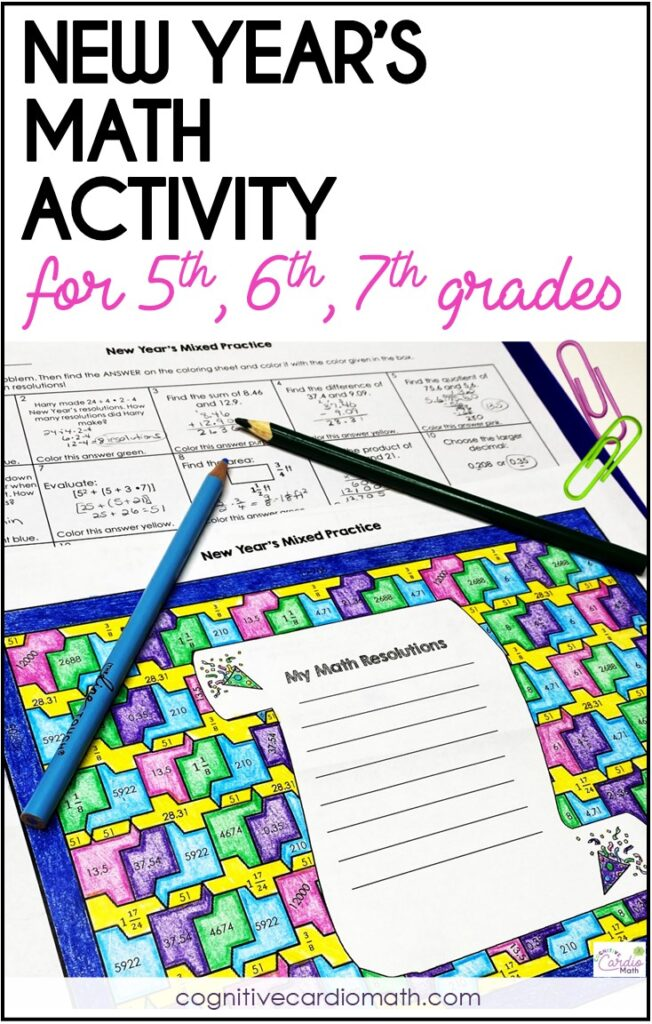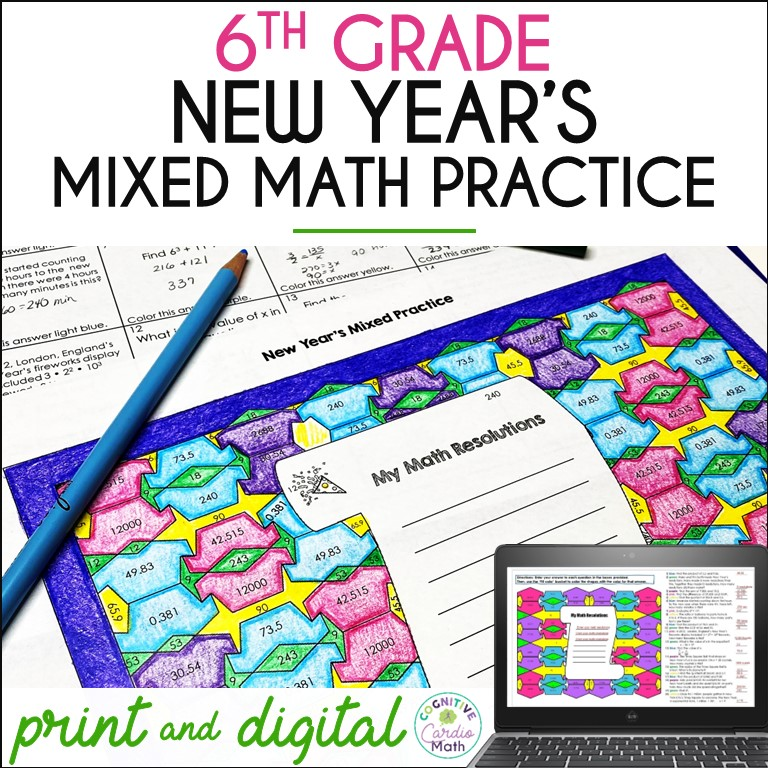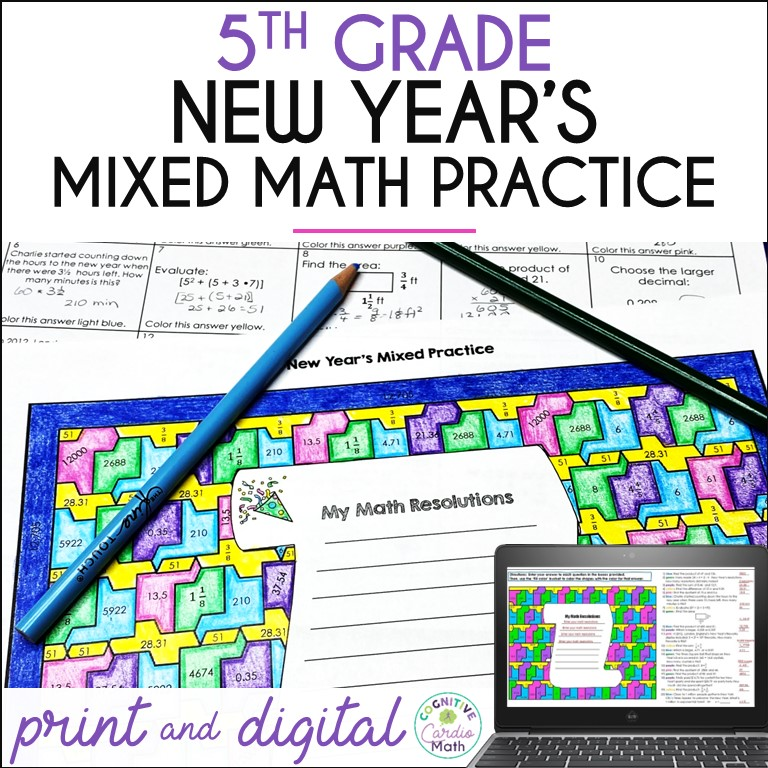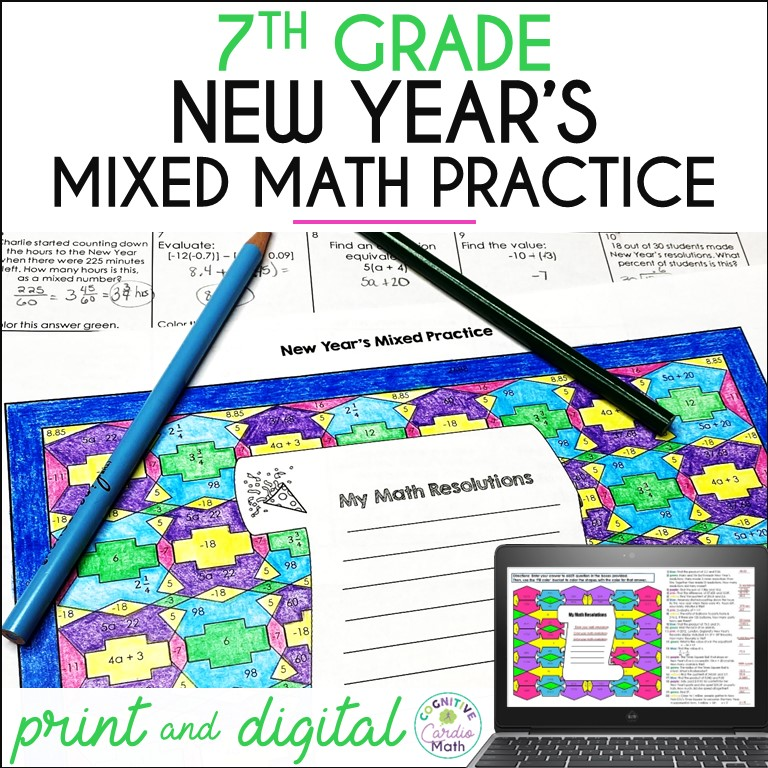# New Year Activities for Middle School Math

Time to ring in the New Year with some engaging new year activities for middle school math!

How do you approach the New Year in your middle school math classes? Do you take time to talk about goals students want to work toward in the New Year?

Or do you talk about the habits students might want to develop to help them be more successful in math class? Or more successful in school in general or even in life overall?

Let’s look at how we might discuss these ideas with our students.## Thinking Back and Looking Forward

Over the years, I found that my 6th grade math students were pretty eager (and if not eager, then at least willing!) to look back at their math ‘performance’ before the winter break and think about whether:

• They’d been doing their best work
• There were particular math things they did well
• There were particular math things that caused them to struggle
• There were math-related things they WISH they had done in that first part of the year

After discussing those ideas, we’d then think about a few math-related goals or resolutions for the New Year and complete some new year activities….like maybe a type of decoration that could be used on a bulletin board to display their goals.

After I read about systems in the book Atomic Habits, we started talking more about systems students could put in place to help them reach their goals. (The idea of systems is a whole different conversation, and you can read about that in the Systems for Student Success blog post).

## New Year Activities for Middle School Math

A few years back, I decided to combine the new year resolution conversation with some practice of math concepts, rather than focusing only on the goals or resolutions.

You’d probably agree that when students come back from winter break, they typically need a little ‘brush up’ work on some math skills. So, I created a combination math review and resolution-making resource for 6th grade math (and then one for 5th and one for 7th too).

This allowed us to have that goal/habit/resolution conversation AND review math concepts all in the same math period.

The 6th grade New Year’s math resource has 20 questions that require students to:

• Multiply fraction by whole number
• Multiply decimal numbers
• Divide decimal numbers
• Evaluate exponents
• Solve word problems
• Solve ratio/proportion
• Find GCF
• Solve one-step equation
• Subtract decimal numbersThe 5th grade New Year’s math activity also has 20 questions, where students:

• Multiply whole numbers
• Divide whole numbers
• Compare decimals
• Subtract decimal numbers
• Multiply decimal numbers
• Divide decimal numbers
• Multiply fraction by whole number
• Multiply fraction by fraction
• Evaluate expressions/OOO
• Find area (multiply fraction & mixed #)
• Find missing exponentAnd the 7th grade math New Year’s review has 20 questions that require students to:

• Solve proportion with fraction
• Find rate
• Find percent of a number
• Solve integer operations problems
• Evaluate expressions using order of operations (w/positive and negative integers)
• Find equivalent expressions (distribute, combine like terms)
• Find circumference
• Convert minutes to hours (as mixed number)Overall, students have loved this reintroduction to math after winter break. They get the chance to brush up on some skills, evaluate their progress so far, and plan for their success in the New Year.

And, as an added bonus, the completed new year’s color by numbers make an awesome bulletin board display!

## EllieWelcome to Cognitive Cardio Math! I’m Ellie, a wife, mom, grandma, and dog ‘mom,’ and I’ve spent just about my whole life in school! With nearly 30 years in education, I’ve taught:

• All subject areas in 4th and 5th grades
• Math, ELA, and science in 6th grade (middle school)

I’ve been creating resources for teachers since 2012 and have worked in the elearning industry for about five years as well!